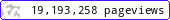•••••MadAsMaths.com :: I.Y.G.B. Practice Papers :: A Level Practice Papers 2019 Spec :: SYNF Practice Papers

I.Y.G.B. SYNF PRACTICE PAPERS

These I.Y.G.B. synoptic further practice papers are suitable for the new A Level Further Pure Mathematics specification, introduced for teaching from 2017.
They do not follow any particular board syllabus; instead they are a synopsis for the Further Pure Mathematics modules.

They will offer good practice for able mathematics students as they will be generally more difficult than the standard "A to R" difficulty of the FP1/FP4 range.

They will also be suitable for students taking A level Further Mathematics from all boards.

All papers have model solutions.

 synf_a.pdfsynf_a_solutions.pdfsynf_b.pdfsynf_b_solutions.pdfsynf_c.pdfsynf_c_solutions.pdfsynf_d.pdfsynf_d_solutions.pdfsynf_e.pdfsynf_e_solutions.pdfsynf_f.pdfsynf_f_solutions.pdfsynf_g.pdfsynf_g_solutions.pdfsynf_h.pdfsynf_h_solutions.pdfsynf_i.pdfsynf_i_solutions.pdfsynf_j.pdfsynf_j_solutions.pdfsynf_k.pdfsynf_k_solutions.pdfsynf_l.pdfsynf_l_solutions.pdfsynf_m.pdfsynf_m_solutions.pdfsynf_n.pdfsynf_n_solutions.pdfsynf_o.pdfsynf_o_solutions.pdfsynf_p.pdfsynf_p_solutions.pdfsynf_q.pdfsynf_q_solutions.pdfsynf_r.pdfsynf_r_solutions.pdfsynf_s.pdfsynf_s_solutions.pdfsynf_t.pdfsynf_t_solutions.pdfsynf_u.pdfsynf_u_solutions.pdfsynf_v.pdfsynf_v_solutions.pdfsynf_w.pdfsynf_w_solutions.pdfsynf_x.pdfsynf_x_solutions.pdfsynf_y.pdfsynf_y_solutions.pdfsynf_z.pdfsynf_z_solutions.pdf